# How to solve (solution) Google's Blockly Future Programmers Game: Maze Level

Google's Blockly Games is a series of educational games that teach programming. It is based on the Blockly library. All code is free and open source. The Maze Game is an introduction to loops and conditionals. It starts simply, but every level is more challenging than the last. The game engine and source is available as an open source project at Github here.

In this article we'll share with you the solution to all 10 levels available in the Maze Game of Blockly.

## Level #1

Stack a couple of 'move forward' blocks together to help me reach the goal.The respective JavaScript code of this level is:

``````moveForward();
moveForward();``````

## Level #2The respective JavaScript code of this level is:

``````moveForward();
turnLeft();
moveForward();
turnRight();
moveForward();``````

## Level #3The respective JavaScript code of this level is:

``````while (notDone()) {
moveForward();
}``````

## Level #4The respective JavaScript code of this level is:

``````while (notDone()) {
moveForward();
turnLeft();
moveForward();
turnRight();
}``````

## Level #5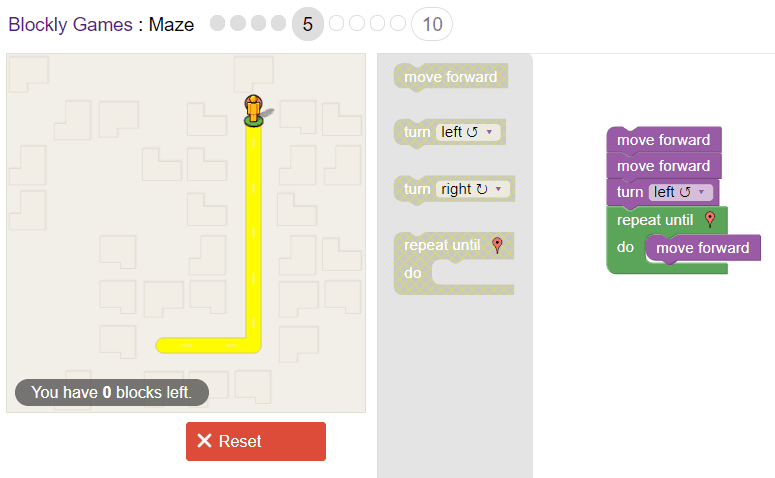The respective JavaScript code of this level is:

``````moveForward();
moveForward();
turnLeft();
while (notDone()) {
moveForward();
}``````

## Level #6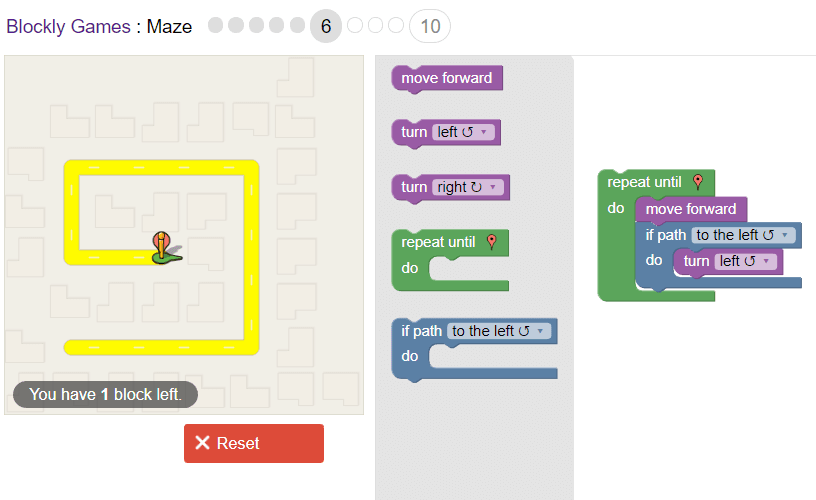The respective JavaScript code of this level is:

``````while (notDone()) {
moveForward();
if (isPathLeft()) {
turnLeft();
}
}``````

## Level #7The respective JavaScript code of this level is:

``````while (notDone()) {
moveForward();
if (isPathRight()) {
turnRight();
moveForward();
}
}``````

## Level #8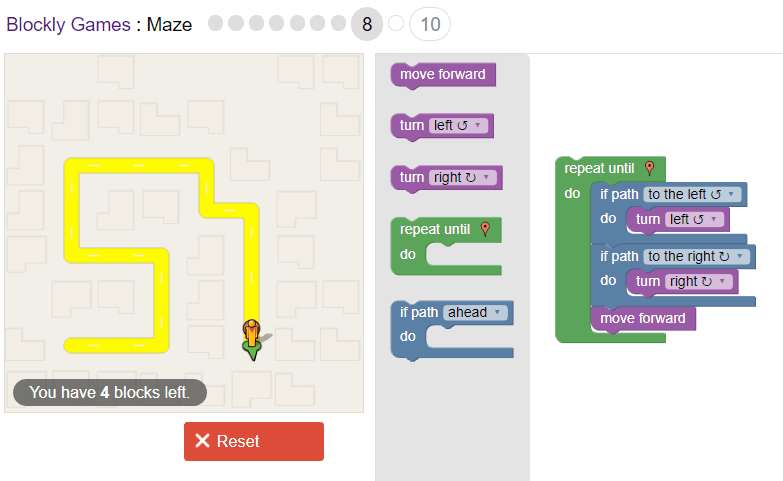The respective JavaScript code of this level is:

``````while (notDone()) {
if (isPathLeft()) {
turnLeft();
}
if (isPathRight()) {
turnRight();
}
moveForward();
}``````

## Level #9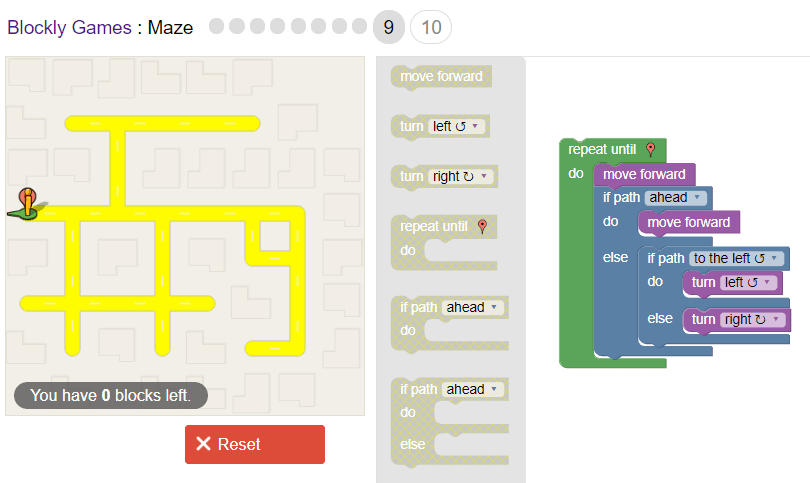The respective JavaScript code of this level is:

``````while (notDone()) {
moveForward();
if (isPathForward()) {
moveForward();
} else {
if (isPathLeft()) {
turnLeft();
} else {
turnRight();
}
}
}``````

## Level #10

Can you solve this complicated maze? Try following the left-hand wall. Advanced programmers only!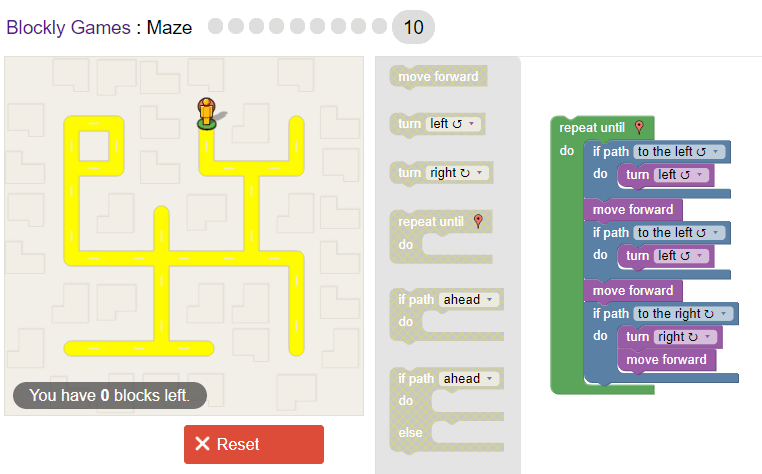The respective JavaScript code of this level is:

``````while (notDone()) {
if (isPathLeft()) {
turnLeft();
}
moveForward();
if (isPathLeft()) {
turnLeft();
}
moveForward();
if (isPathRight()) {
turnRight();
moveForward();
}
}``````

Happy coding !